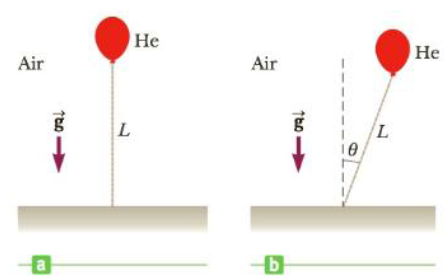Chapter 13, Problem 71AP

Chapter
Section
Textbook Problem

A light balloon filled with helium of density 0.179 kg/m3 is tied to a light string of length L = 3.00 m. The string is tied to the ground, forming an “inverted” simple pendulum (Fig. P13.71a). If the balloon is displaced slightly from equilibrium, as in Figure P13.71b, (a) show that the motion is simple harmonic and (b) determine the period of the motion. Take the density of air to be 1.29 kg/m3. Hint: Use an analogy with the simple pendulum discussed in the text, and see Topic 9.Figure P13.71

(a)

To determine
The motion is harmonic.

Explanation

Given info: The length of the string is 3.00m . The density of helium is 0.179kgm3 . The density of air is 1.29kgm3 .

Explanation:

The system is shown in the diagram

• B is the buoyant force on the balloon
• T is the tension force
• mg is the gravitational force

The net restoring force will be,

F=Bsinθ+mgsinθ (1)

For small angles,

sinθ=θ=sL (2)

The gravitational force on the balloon will be,

mg=ρHeVg (3)

• <

b)

To determine
The period of the motion.

Still sussing out bartleby?

Check out a sample textbook solution.

See a sample solution

The Solution to Your Study Problems

Bartleby provides explanations to thousands of textbook problems written by our experts, many with advanced degrees!

Get Started